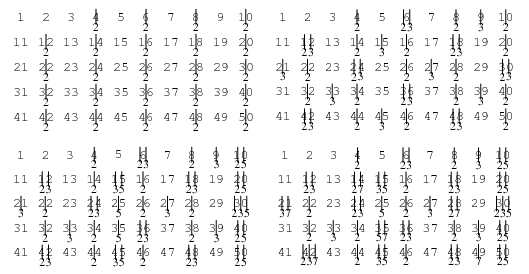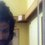# Sieve of EratosthenesAn algorithm for making tables of primes. Sequentially write down the integers from 2 to the highest number n you wish to include in the table. Cross out all numbers >2 which are divisible by 2 (every second number). Find the smallest remaining number >2. It is 3. So cross out all numbers >3 which are divisible by 3 (every third number). Find the smallest remaining number >3. It is 5. So cross out all numbers >5 which are divisible by 5 (every fifth number).

Continue until you have crossed out all numbers divisible by $\left\lfloor \sqrt { n } \right\rfloor$, where $\left\lfloor x \right\rfloor$ is the floor function. The numbers remaining are prime. This procedure is illustrated in the above diagram which sieves up to 50, and therefore crosses out composite numbers up to $\left\lfloor \sqrt { 50 } \right\rfloor =7$. If the procedure is then continued up to n, then the number of cross-outs gives the number of distinct prime factors of each number.Note by Crazy Singh
7 years, 4 months ago

This discussion board is a place to discuss our Daily Challenges and the math and science related to those challenges. Explanations are more than just a solution — they should explain the steps and thinking strategies that you used to obtain the solution. Comments should further the discussion of math and science.

When posting on Brilliant:

• Use the emojis to react to an explanation, whether you're congratulating a job well done , or just really confused .
• Ask specific questions about the challenge or the steps in somebody's explanation. Well-posed questions can add a lot to the discussion, but posting "I don't understand!" doesn't help anyone.
• Try to contribute something new to the discussion, whether it is an extension, generalization or other idea related to the challenge.

MarkdownAppears as
*italics* or _italics_ italics
**bold** or __bold__ bold
- bulleted- list
• bulleted
• list
1. numbered2. list
1. numbered
2. list
Note: you must add a full line of space before and after lists for them to show up correctly
paragraph 1paragraph 2

paragraph 1

paragraph 2

[example link](https://brilliant.org)example link
> This is a quote
This is a quote
    # I indented these lines
# 4 spaces, and now they show
# up as a code block.

print "hello world"
# I indented these lines
# 4 spaces, and now they show
# up as a code block.

print "hello world"
MathAppears as
Remember to wrap math in $$ ... $$ or $ ... $ to ensure proper formatting.
2 \times 3 $2 \times 3$
2^{34} $2^{34}$
a_{i-1} $a_{i-1}$
\frac{2}{3} $\frac{2}{3}$
\sqrt{2} $\sqrt{2}$
\sum_{i=1}^3 $\sum_{i=1}^3$
\sin \theta $\sin \theta$
\boxed{123} $\boxed{123}$